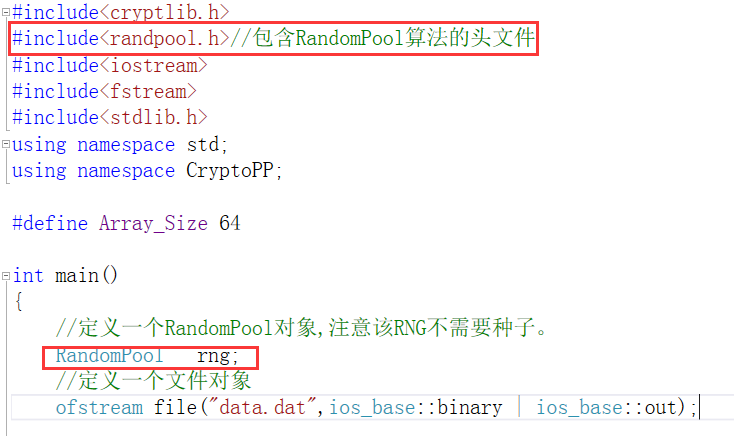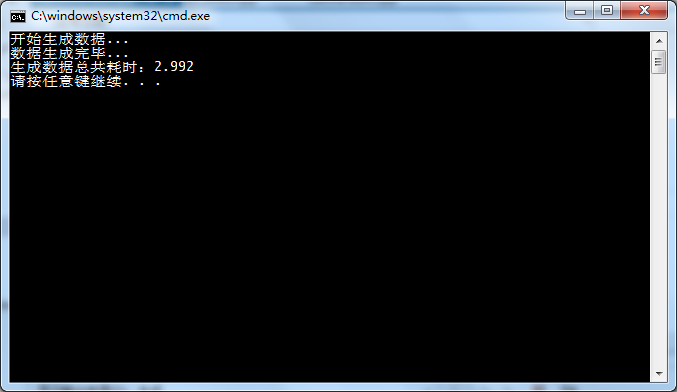## CryptoPP的RandomPool算法的使用_柳亓的博客-程序员宅基地_randompoolhttps://blog.csdn.net/Lunar_Queen/article/details/81542372以及其帮助文档。``````#include<cryptlib.h>
#include<randpool.h>//包含RandomPool算法的头文件
#include<iostream>
#include<fstream>
#include<stdlib.h>
using namespace std;
using namespace CryptoPP;

#define Array_Size 64

int main()
{
//定义一个RandomPool对象,注意该RNG不需要种子。
RandomPool   rng;
//定义一个文件对象
ofstream file("data.dat",ios_base::binary | ios_base::out);

byte output[Array_Size];

//产生1Gbits的数据。每调用一次随机数发生器，产生8*64=512bits的数据。
//1Gbits = 1000*1000*1000。
//1000*1000*1000/512 = 1953125。

cout << "开始生成数据..." << endl;
clock_t start = clock();

for(int i=0; i < 1953125 ; ++i)
{
rng.GenerateBlock(output,Array_Size);
file.write(reinterpret_cast<const char*>(output),Array_Size);
}
clock_t end = clock();
cout << "数据生成完毕..." << endl;

double  duration;
duration = (double)(end - start) / CLOCKS_PER_SEC;
cout << "生成数据总共耗时：" << duration << endl;

file.close();

return 0;
}
``````